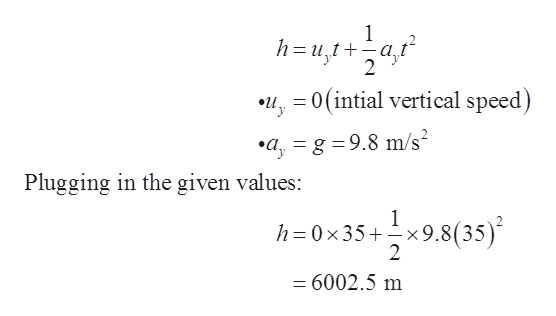# An airplane is flying horizontally with speed 1300 km/h (360 m/s ) when an engine falls off. Neglecting air resistance, assume it takes 35 s for the engine to hit the groundA)Find the altitude of the airplane. (Use g=9.8m/s2.).B)Find the horizontal distance the airplane engine falls.C)If the airplane somehow continues to fly as if nothing had happened, where is the engine relative to the airplane at the moment the engine hits the ground?

Question
14 views

An airplane is flying horizontally with speed 1300 km/h (360 m/s ) when an engine falls off. Neglecting air resistance, assume it takes 35 s for the engine to hit the ground

A)Find the altitude of the airplane. (Use g=9.8m/s2.).

B)Find the horizontal distance the airplane engine falls.

C)If the airplane somehow continues to fly as if nothing had happened, where is the engine relative to the airplane at the moment the engine hits the ground?

check_circle

Step 1

Given data:

Horizontal speed of the plan, ux = 1300 km/h = 360 m/s

Time taken to reach the ground, t = 35 s

Step 2

(a)

The altitude of the plan can be calculated by the second equation of the kinematics:help_outlineImage Transcriptionclose1 h=u1+af 2 2u0(intial vertical speed) ayg =9.8 m/s2 Plugging in the given values: 1 h=0x35+x9.8(35) 2 =6002.5 m fullscreen
Step 3

(b)

The horizontal distance of the airplane:

...

### Want to see the full answer?

See Solution

#### Want to see this answer and more?

Solutions are written by subject experts who are available 24/7. Questions are typically answered within 1 hour.*

See Solution
*Response times may vary by subject and question.
Tagged in

### Other